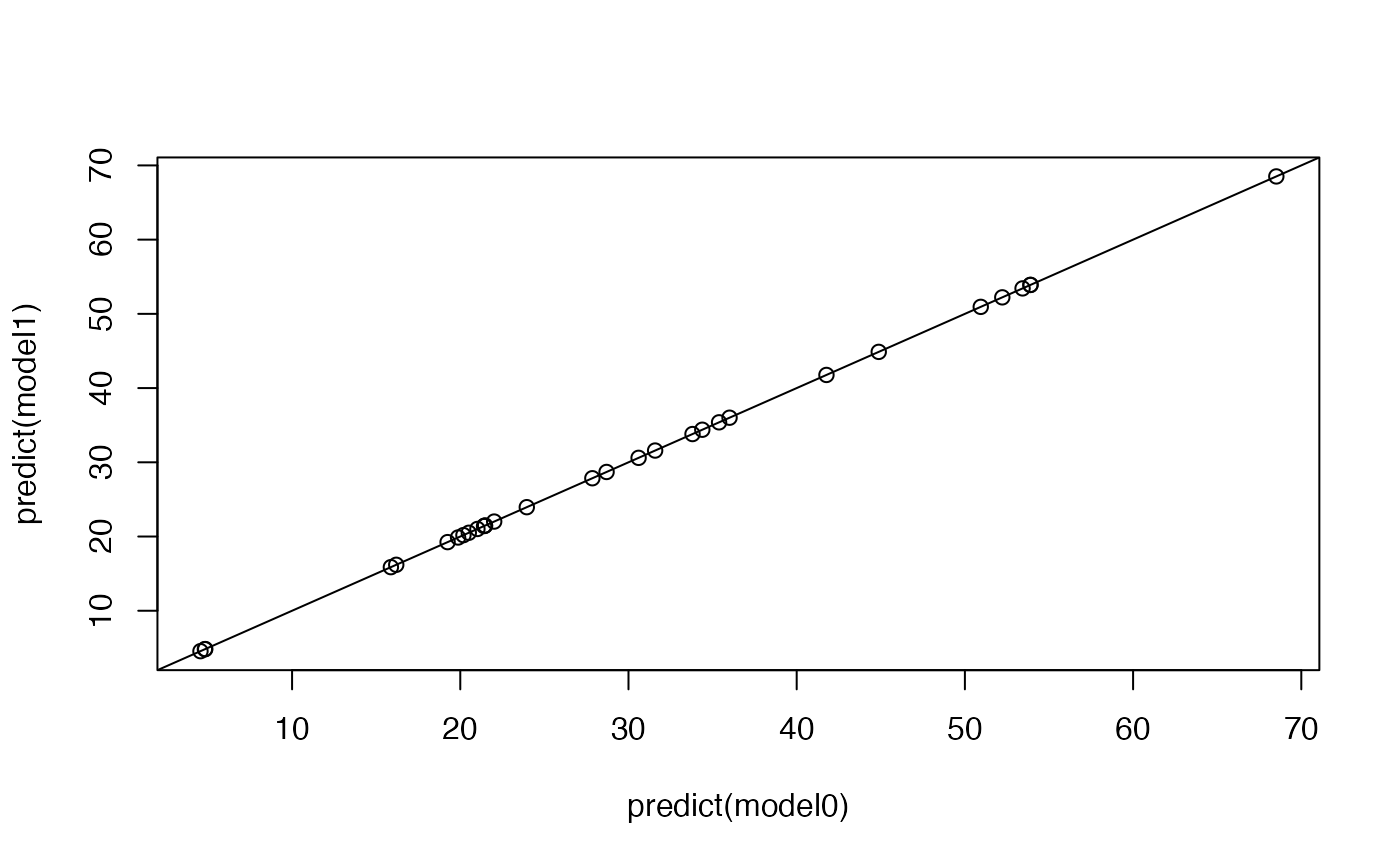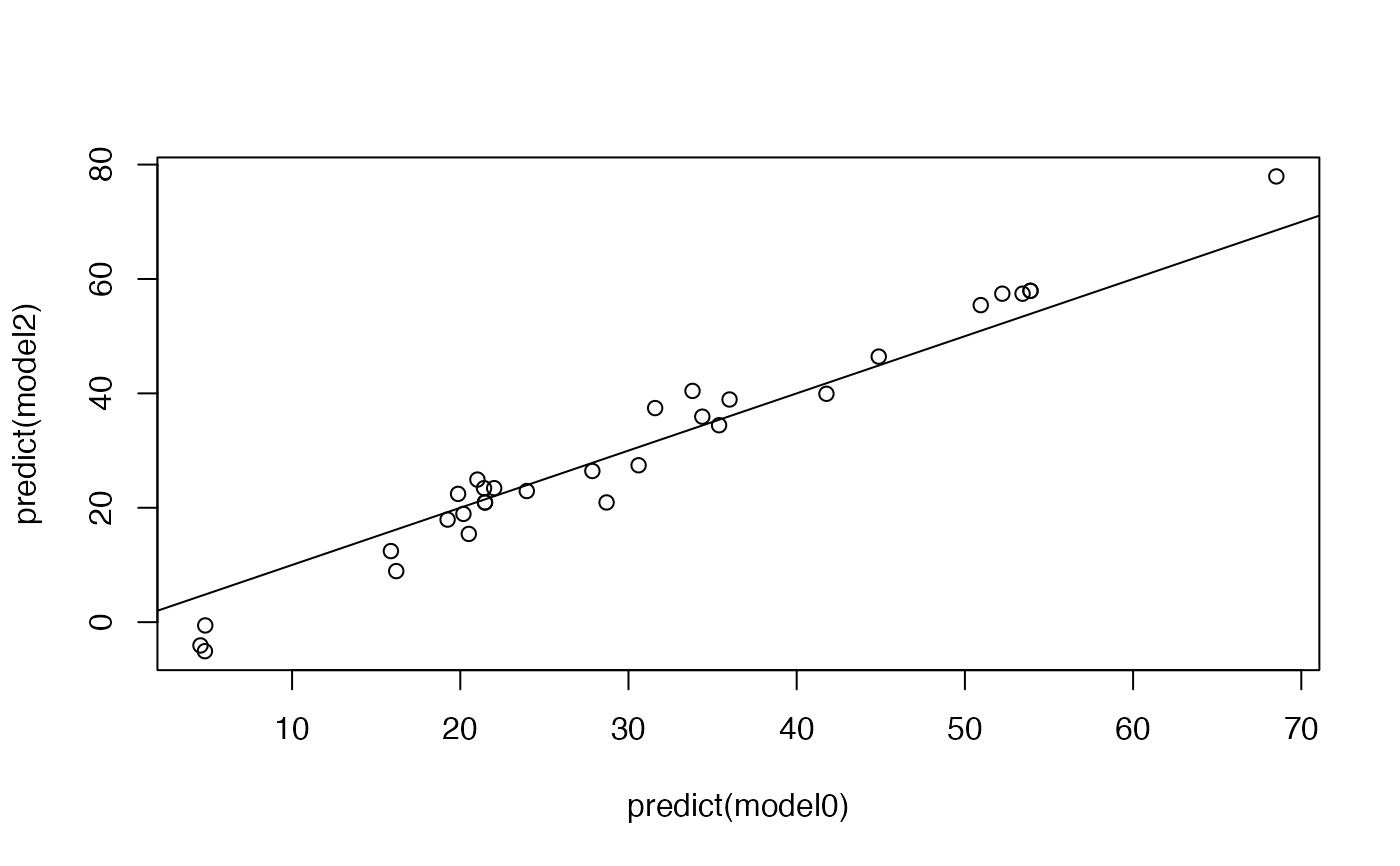Refits a model with a specified set of coefficients.

## Usage

fix.coef(model, beta = NULL)

## Arguments

model

An R model, e.g., produced by lm or glm

beta

A numeric vector with length(coef) model coefficients. If the vector is not named, the coefficients should be given in the same order as in coef(model). If the vector is named, the procedure attempts to match on names.

## Value

An updated R model object

## Details

The function calculates the linear predictor using the new coefficients, and reformulates the model using the offset argument. The linear predictor is called offset, and its coefficient will be 1 by definition. The new model only fits the intercept, which should be 0 if we set beta = coef(model).

## Author

Stef van Buuren, 2018

## Examples

model0 <- lm(Volume ~ Girth + Height, data = trees)
formula(model0)
#> Volume ~ Girth + Height
#> <environment: 0x55589a85ede0>
coef(model0)
#> (Intercept)       Girth      Height
#> -57.9876589   4.7081605   0.3392512
deviance(model0)
#>  421.9214

# refit same model
model1 <- fix.coef(model0)
formula(model1)
#> Volume ~ 1
#> <environment: 0x55589a85ede0>
coef(model1)
#>  (Intercept)
#> 1.125519e-14
deviance(model1)
#>  421.9214

# change the beta's
model2 <- fix.coef(model0, beta = c(-50, 5, 1))
coef(model2)
#> (Intercept)
#>   -62.07097
deviance(model2)
#>  1098.984

# compare predictions
plot(predict(model0), predict(model1))
abline(0, 1)plot(predict(model0), predict(model2))
abline(0, 1)# compare proportion explained variance
cor(predict(model0), predict(model0) + residuals(model0))^2
#>  0.94795
cor(predict(model1), predict(model1) + residuals(model1))^2
#>  0.94795
cor(predict(model2), predict(model2) + residuals(model2))^2
#>  0.9228528

# extract offset from constrained model
summary(model2\$offset)
#>    Min. 1st Qu.  Median    Mean 3rd Qu.    Max.
#>   57.00   82.00   87.00   92.24  102.25  140.00

# it also works with factors and missing data
model0 <- lm(bmi ~ age + hyp + chl, data = nhanes2)
model1 <- fix.coef(model0)
model2 <- fix.coef(model0, beta = c(15, -8, -8, 2, 0.2))